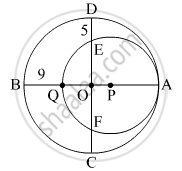SSC (Marathi Semi-English) 10thMaharashtra State Board
Share

# In the Given Figure , Two Circles with Centres O and P Are Touching Internally at Point A. If Bq = 9, De = 5, Complete the Following Activity to Find the Radii of the Circles. - SSC (Marathi Semi-English) 10th - Geometry

ConceptSurface Area of a Combination of Solids

#### QuestionIn the given figure , two circles with centres O and P are touching internally  at point A. If BQ = 9, DE = 5, complete the following activity to find the radii of  the circles.

#### Solution

Let the radius of the bigger circle be R and that of smaller circle be r.
OA, OB, OC and OD are the radii of the bigger circle.
∴ OA = OB = OC = OD = R
PQ = PA = r
OQ = OB − BQ = R - 9

OE = OD − DE = R - 5

As the chords QA and EF of the circle with centre P intersect in the interior of the circle, so by the property of internal division of two chords of a circle,
OQ × OA = OE × OF R - 9 x r = R - 5 x R - 5    .....(∵ OE = OF)
R2 − 9R = R2 − 10+ 25
R = 25

AQ = 2r = AB − BQ                      (∵AB = 2R)
2r = 50 − 9 = 41
r = $\frac{41}{2}$ = 20.5

Is there an error in this question or solution?

#### APPEARS IN

Solution In the Given Figure , Two Circles with Centres O and P Are Touching Internally at Point A. If Bq = 9, De = 5, Complete the Following Activity to Find the Radii of the Circles. Concept: Surface Area of a Combination of Solids.
S